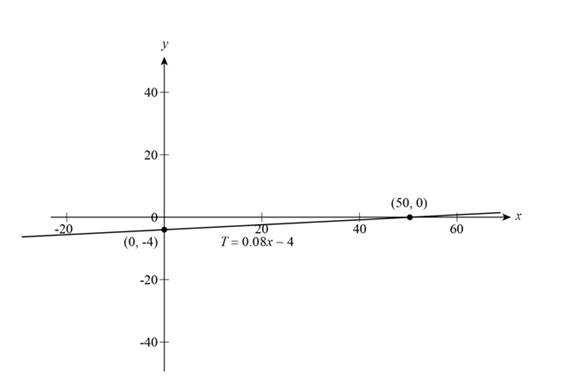# The temperature of soil at a depth of 1 m .### Precalculus: Mathematics for Calcu...

6th Edition
Stewart + 5 others
Publisher: Cengage Learning
ISBN: 9780840068071### Precalculus: Mathematics for Calcu...

6th Edition
Stewart + 5 others
Publisher: Cengage Learning
ISBN: 9780840068071

#### Solutions

Chapter 1, Problem 21T

(a)

To determine

## The temperature of soil at a depth of 1 m .

Expert Solution

The temperature of soil at a depth of 1m is 4C .

### Explanation of Solution

Given:

The equation for temperature is T=0.08x4 , x is depth and T is temperature and the value of depth is 1m .

The value of x is 100 in centimeters.

Calculation:

Substitute 100 for x in the given equation to get the value of temperature,

T=0.08(100)4=84=4

Thus, the temperature of soil at a depth of 1m is 4°C .

(b)

To determine

Expert Solution

### Explanation of Solution

Calculation:

The given linear equation is T=0.08x4 .

The above equation is linear so that graph of equation is a line and to draw line there should be two points.

Substitute 0 for T to get x intercept,

0=0.08x4x=40.08x=4008x=50

Substitute 0 for x to get T-intercept,

T=0.08(0)4T=4

From the intercepts line passes through two points (50,0) , (0,4) . So, the graph is shown below,Figure (1)

Figure (1) shows the graph of linear equation T=0.08x4 which represents a line.

(c)

To determine

### The purpose of slope, x-intercept and T-intercept of the graph of equation T=0.08x−4 .

Expert Solution

The slope increases temperature of 0.08°C for each one-centimeter increase in depth, the x-intercept is the depth at which the temperature is 0°C , and the T-intercept is the temperature at ground level.

### Explanation of Solution

The given linear equation is T=0.08x4 .

In the above equation temperature is T at ground level and x-intercept is depth of soil that is given and temperature T varies according to x values because it is dependent on the x.

The T-intercept is 4 when depth x is zero which give the value of temperature at ground level shown in above Figure (1).

Thus, the slope increases temperature of 0.08°C for each one-centimeter increase in depth, the x-intercept is the depth at which the temperature is 0°C , and the T-intercept is the temperature at ground level.

### Have a homework question?

Subscribe to bartleby learn! Ask subject matter experts 30 homework questions each month. Plus, you’ll have access to millions of step-by-step textbook answers!